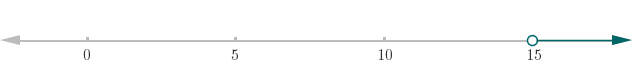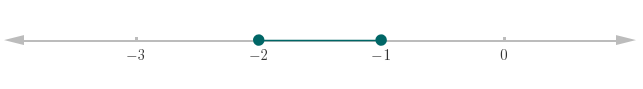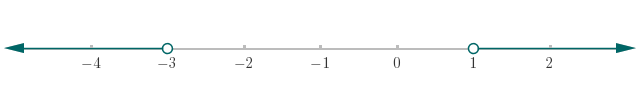Inequalities homework Help at TutorEye

## Top Questions

nly shows one number but i can send all the numbers as pdf
View More

of x, y, and r ii) at least 2 possible angles for which the following is true Cos (Ø)= -2/3
View More

volume of carbon tetrachloride would be produced by this reaction if 8.91 cm3 of methane were consumed? Also, be sure your answer has a unit symbol, and is rounded to the correct number of significant digits.
View More

ake any payments for 2 years, how much will he owe on this debt in 2 years by compounding continuously?
View More

## Inequalities Sample Questions

Question 1: Solve for unknown variable x, and write the answer using interval notation.Answer:Explanation:  Expand the bracket. And, rearrange the terms to solve forQuestion 2: Solve for q.Answer:Explanation:  Rearrange the terms to solve for q.

Question 3: Solve for r, and write the solution using interval notation.Answer:Explanation:  Expand the bracket. And, rewrite the terms to solve for.

Question 4: Solve for unknown variable x, and write the solution using interval notation.Answer:Explanation:  Subtract 2 from all parts of inequality.

Question 5: Solve for unknown variable x, and write the solution using interval notation.Answer:Explanation:  Add 3 to all parts of inequality.

Question 6: Solve for y.Answer:Explanation:  Add 3 to both sides of inequality.

Question 7: Solve for z, and write the answer using interval notation.Answer:Explanation:  Rearrange the terms to isolate the z.

Question 8: Solve for a. And, show the solution on the number line.Answer:Explanation:  Rearrange the terms to isolate the a.

Question 9: Solve for b. And, show the solution on the number line.Answer:Explanation:  Add 4 to all sides of the inequality.

Question 10: Solve for x, write the interval solution. And, show the solution on the number line.orAnswer:Explanation:  Solve each inequality.